# Almost Periodic Function

(redirected from Almost periodic functions)

## Almost Periodic Function

a function whose value is approximately repeated when its argument is increased by properly selected constants (the almost periods). More precisely, a continuous function f(x) defined for all real values of 5 x is called almost periodic if for every > 0 there exists an l = l (∊) such that in every interval of length l on the x-axis at least one number τ = τ(∊) can be found for which the inequality ǀf (x + τ) – f(x)ǀ < ∊ is satisfied for all x. The numbers τ are called the almost periods of the function f(x). Periodic functions are special cases of almost periodic functions; simple examples of almost periodic functions that are not periodic can be obtained by adding periodic functions with incommensurable periods—for example, cos x + cos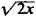.

The following are some important properties of almost periodic functions:

(1) An almost periodic function is bounded and uniformly continuous on the entire x-axis.

(2) The sum and product of a finite number of almost periodic functions is an almost periodic function.

(3) The limit of a uniformly convergent sequence of almost periodic functions is an almost periodic function.

(4) Every almost periodic function has a mean value (over the entire x-axis):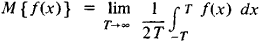(5) To every almost periodic function we can associate a Fourier series: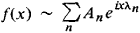where λ1, λ2, …, λn, … can be any sequence of distinct real numbers and

An = M {f (x)enx}

(6) The Parseval equality: for every almost periodic function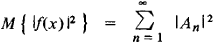(7) Uniqueness theorem: if f(x) is a continuous almost periodic function and if for all real λ

M {f (x)eiλx} = 0

then f(x) ≡ 0. In other words, a Fourier series uniquely determines an almost periodic function.

(8) Approximation theorem: for every ∊ > 0, there exists a finite trigonometric polynomial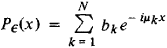(where μκ is a real number) such that the inequality ǀf(x) – P (x)ǀ < ∊ is satisfied for all values of x; conversely, every function f(x) with this property is an almost periodic function.

The first construction of almost periodic functions was given by the Danish mathematician H. Bohr in 1923. Even earlier, in 1893, the Latvian mathematician P. Bohl studied a special case of almost periodic functions—quasi-periodic functions. A new construction of the theory of almost periodic functions was provided by N. N. Bogoliubov in 1930. The theory of almost periodic functions was generalized to include discontinuous functions first by V. V. Stepanov in 1925 and subsequently by H. Weyl and A. S. Besicovitch. A generalization of a different kind was given by the Soviet mathematician B. M. Levitan in 1938.

### REFERENCES

Bohr, H. Pochti periodicheskie funktsii. Moscow-Leningrad, 1934. (Translated from German.)
Levitan, B. M. Pochti-periodicheskie funktsii. Moscow, 1953.
References in periodicals archive ?
showed sufficient conditions to ensure the existence and uniqueness of mild solution for (2) in the following classes of vector-valued function spaces: periodic functions, asymptotically periodic functions, pseudo periodic functions, almost periodic functions, asymptotically almost periodic functions, pseudo almost periodic functions, almost automorphic functions, asymptotically almost automorphic functions, pseudo almost automorphic functions, compact almost automorphic functions, asymptotically compact almost automorphic functions, pseudo compact almost automorphic functions, S-asymptotically [omega]-periodic functions, decay functions, and mean decay functions.
Almost periodic functions, which are an important generalization of periodic functions, were introduced into the field of mathematics by Bohr [1, 2].
where [a.sub.ij], [b.sub.ij], [[beta].sub.im], [[gamma].sub.im] : R [right arrow] (0, +[infinity]) and [[tau].sub.im] : R [right arrow] [R.sub.+] are continuous almost periodic functions with i,j = 1, 2,..., n, m = 1, 2,..., l.
We regard the direct sum of [P.sub.[omega]](X) and [C.sub.0](X) as the space of asymptotically periodic functions A[P.sub.[omega]](X), the direct sum of AP(X) and [C.sub.0](X) as the space of asymptotically almost periodic functions AAP(X), the direct sum of [AA.sub.c](X) and [C.sub.0](X) as the space of asymptotically compact almost automorphic functions, and the direct sum of AA(X) and [C.sub.0](X) as the space of asymptotically almost automorphic functions.
The concept of almost periodic functions on time scales in [R.sup.n] was proposed and investigated in [15, 16].
(H1) [B.sup.kl.sub.ij], [C.sup.kl.sub.ij], and [I.sub.ij] are continuous almost periodic functions, where i, k = 1, 2, ..., m and j, l = 1, 2, ..., n.
The case [phi](u) = [u.sup.r], 1 [less than or equal to] r [less than or equal to] 2, is discussed in our papers quoted above, leading to the spaces A[P.sub.r](R, C), r [member of] [1, 2], which constitute a scale of function/spaces starting with A[P.sub.1] (R, C) = the space of Poincare of almost periodic functions (actually a Banach algebra, see Corduneanu , for instance), and ending with the Besicovitch space [[beta].sub.2](R, C).
The theory of almost periodic functions has been developed in connection with problems of differential equations, stability theory, dynamical systems and many others.
where [tau](t) and p(t) are almost periodic functions on R, m > 1, and a, b, and c are constants.
It will be assumed that [gamma], [a.sub.1], [a.sub.2], [tau] : R [g.sup.+] [0, + [infinity]) are continuous almost periodic functions. Let [R.sub.+] denote the nonnegative real number space, let C = C((-[[tau].sup.+], 0], R) be the continuous functions space equipped with the usual supremun norm [parallel] * [parallel], and let [C.sub.+] = C((-[[tau].sup.+], 0], [R.sub.+]).

Site: Follow: Share:
Open / Close